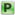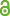Hauptmenü
• Autor
• Berkes, István
• TitelStrong approximation of the St. Petersburg game
• Datei
• DOI10.1080/02331888.2016.1269476
• Erschienen inA Journal of Theoretical and Applied Statistics
• Band51
• Erscheinungsjahr2017
• Heft1
• Seiten3-10
• LicenceCC BY
• ISSN1029-4910
• Zugriffsrechte• AbstractLet $$X, X_1 , X_2 , . . .$$ be i.i.d. random variables with $$P(X = 2^k) = 2^{-k} (k ∈ \mathbb{N}$$ and let $$S_n = ∑^n_{k=1} X_k$$ . The properties of the sequence $$S_n$$ have received considerable attention in the literature in connection with the St. Petersburg paradox (Bernoulli 1738). Let $$\{Z(t), t ≥ 0\}$$ be a semistable Lévy process with underlying Lévy measure $$∑_{k∈\mathbb{Z}} 2^{−k} δ_{2^k}$$ . For a suitable version of $$(X_k)$$ and $$Z(t)$$ , we prove the strong approximation $$S_n = Z(n) + O(n_{5/6+ε})$$a.s. This provides the first example for a strong approximation theorem for partial sums of i.i.d. sequences not belonging to the domain of attraction of the normal or stable laws.